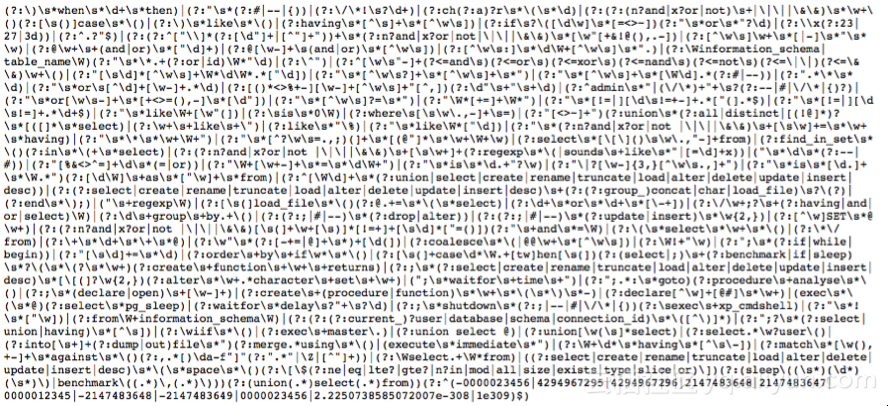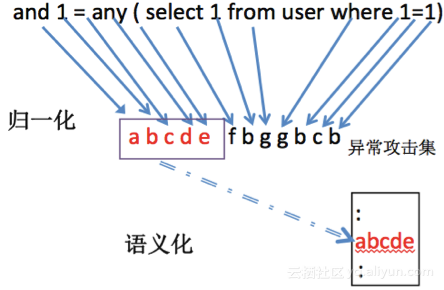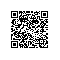# 大道至简：智能语义检测的武林

“智能语义检测”这门武艺的江湖故事，要从它的前辈“基于规则的检测引擎”开始说起。

10几年里，基于规则的引擎一统江湖。直到现在，江湖上大多数的WAF是基于规则的WAF。其原理是每一个会话都要经过一系列的安全检测，每一项检测都由一个或多个检测规则组成，匹配了检测规则，请求就会被认为非法而拒绝。www.vuln.com/?id=1’ union select version() from dual

union\s+select\s+version\s+from\s+dua

\s+的作用是匹配一个或多个不可见字符，如空格、换行等符号。显然的，熟悉SQL语句的攻击者，可以利用一些数据库特性绕过这个正则的检查，例如：用注释符/*11*/替换空格、利用注释符号--%0替换空格，根据这个特性，完善后的正则表达式可以写为：

union(\s+|/\*[\s\S]*\*/|--[\s\S]*?\x0a)select(\s+|/\*[\s\S]*\*/|--[\s\S]*?\x0a)version(\s+|/\*[\s\S]*\*/|--[\s\S]*?\x0a)from(\s+|/\*[\s\S]*\*/|--[\s\S]*?\x0a)dual

union(\s+|/\*[\s\S]*\*/|--[\s\S]*?\x0a)select(\s+|/\*[\s\S]*\*/|--[\s\S]*?\x0a)version(\s+|/\*[\s\S]*\*/|--[\s\S]*?\x0a|)(\s+|/\*[\s\S]*\*/|--[\s\S]*?\x0a)from(\s+|/\*[\s\S]*\*/|--[\s\S]*?\x0a)dual1、“大道至简！”是攻击

2、“至繁归于至简！”因为场景和顺序不对，不是攻击

3、通过机器学习，能分辨出“大道无为”是“大道至简”的变体(select|from|\band|\bor|\bxor|=|,|;)[\s\+\()*?(sleep[\s\+]*?\(|version[\s\+]*?\(|pg_sleep[\s\+]*?\(|extractvalue[\s\+]*?\(|updatexml[\s\+]*?\(|dbms_pipe.receive_message\(|st_latfromgeohash\(|st_longfromgeohash[\s\+]*?\(|analyse[\s\+]*?\(|gtid_subset[\s\+]*?\(|gtid_subtract\(|st_pointfromgeohash\(|convert[\s\+]*?\(|md5[\s\+]*?\(|count[\s\+]*?\(|char[\s\+]*?\(|benchmark[\s\+]*?\(|hex[\s\+]*?\(|@@version|db_name[\s\+]*?\(|user[\s\+]*?\(|cast[\s\+]*?\(|concat[\s\+]*?\(|unhex[\s\+]*?\(|floor[\s\+]*?\(|length[\s\+]*?\(|ascii[\s\+]*?\(|substring[\s\+]*?\(|substr[\s\+]*?\(|substring_index[\s\+]*?\(|instr[\s\+]*?\(|left[\s\+]*?\(|right[\s\+`]\()### 制胜之招：无影https://www.aliyun.com/product/yundunall?spm=5176.8142029.388261.22.83Ye8G